# C# Bitwise Operators - c# - c# tutorial - c# net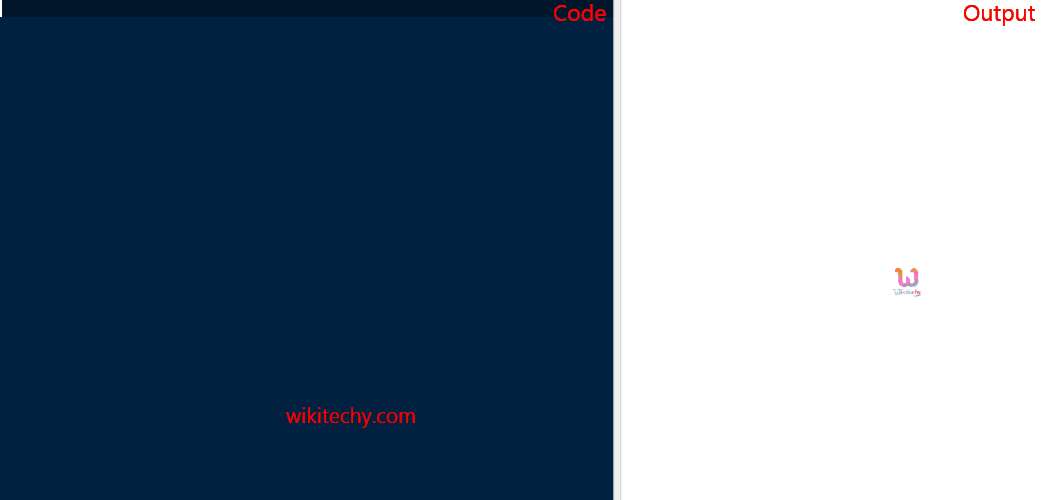C# Bitwise Operator

## What are the types of bitwise operator in C# ?

• These operators are used to perform bit operations.
• Binary numbers are native to computers. Binary, octal, decimal, or hexadecimal symbols are only notations of the same number.
• Bitwise operators work with bits of a binary number.
• Bitwise operators are rarely used in higher level languages like C#.
• & (bitwise AND),
• | (bitwise OR),
• ~ (bitwise NOT),
• ^ (XOR),
• << (left shift) and
• >> (right shift).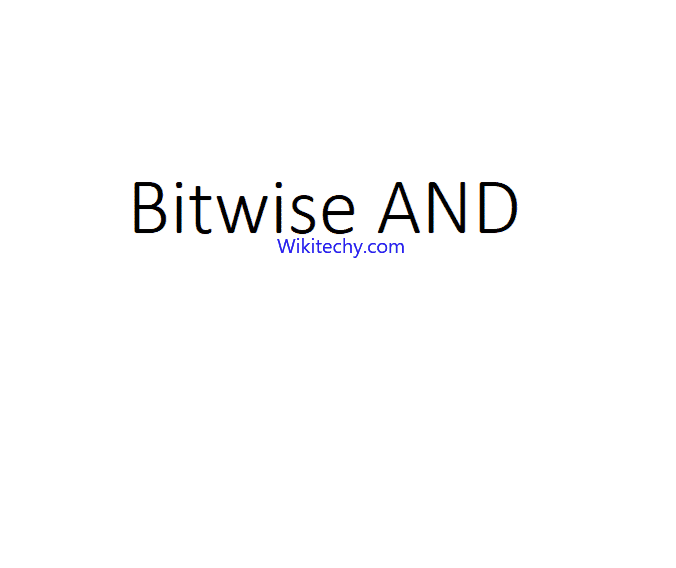Bitwise Operator

NOTE: In general, the bitwise NOT operator covert all the 0’s to 1’s.The bitwise left shift "x<<1" means the bit values are left shifted in one place.

## Code Explanation: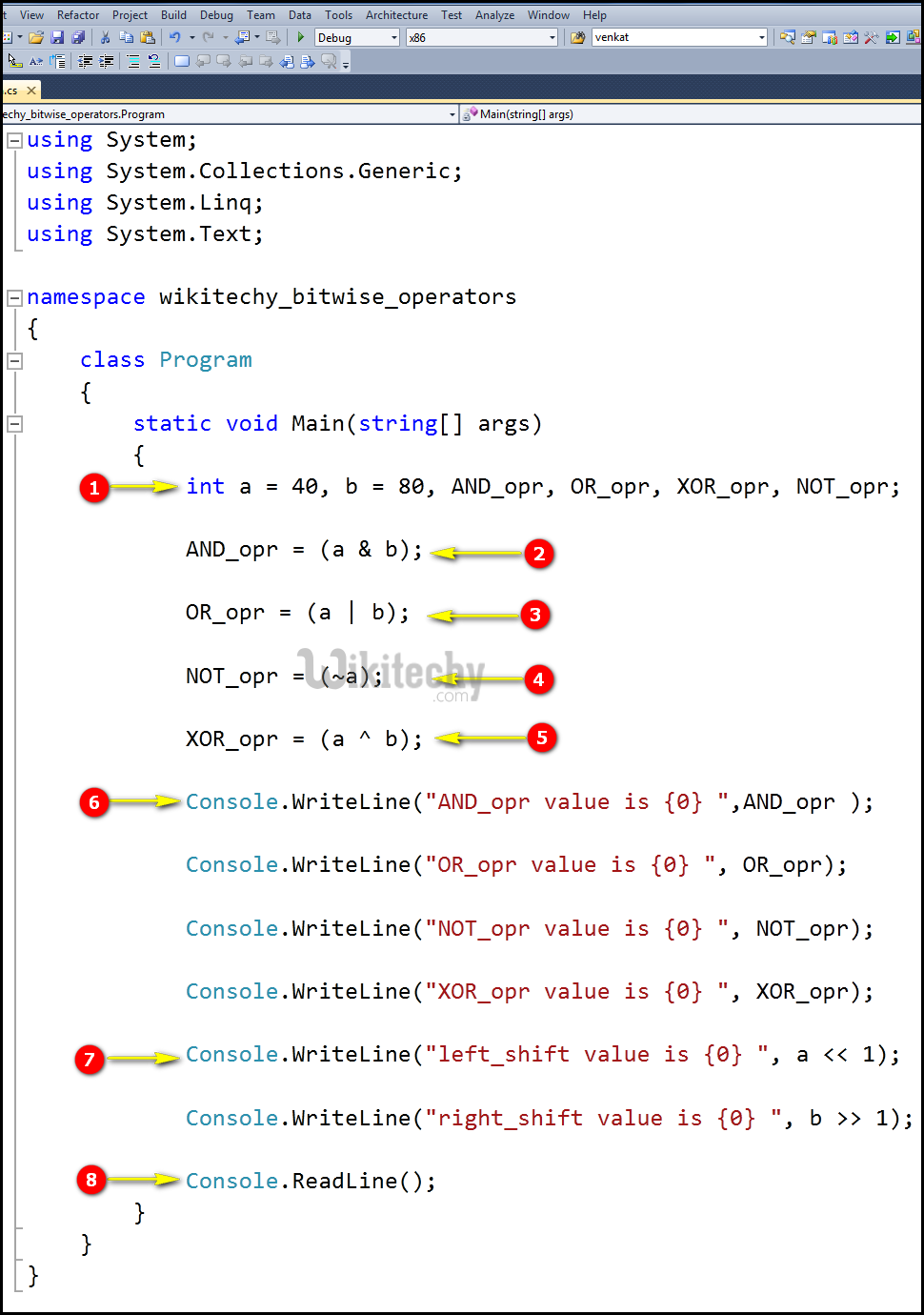1. Here in this statement we initialize the value of the variable "a=40 and b=80" and defined the variables AND_opr, OR_opr, XOR_opr & NOT_opr.
2. In this statement we are using the bitwise logical operator AND (&) it works in such a way as multiplication operation, here the first value will be converted into binary format. For example, here we have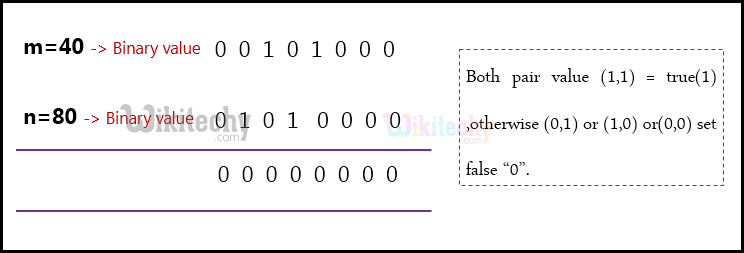now on checking each digit with their binary value the common values are set as"1" remaining are set as "0", so we get the output as "00000000" which is the decimal value of "0" that value will be store in the variable AND_opr.
3. In this statement we are using the bitwise logical operator OR( | ) and it works such a way as addition operation, here the first value will be converted into binary format .We have a=40 binary value as "00101000" and b="80" binary value as "01010000" , now on checking each digits with their value if any digits is "1" it sets as "1" ,if both the digits are common means it sets as "0" ,so you get the output as "01111000" which is the decimal value of "120" that value will be store in the variable OR_opr.
4. In this statement, we are using the bitwise logical operator NOT (~) it works in such a way like the first value will be converted into binary format and it takes the 2’s complement for the value that will be store in this variable NOT_opr.
5. In this statement, we are using the bitwise logical operator XOR (^) it works in such a way like, the first value will be converted into binary format. Here we have a=40 binary value as "00101000" and b="80" binary value as "01010000" now on checking each digit with their value if any digits is "1" means it sets as"1", if both the digits are "0" (or) "1" means it sets as "0" .so you get the output as "01111000" and the decimal value "68" that value will be store in the variable OR_opr.
6. Here we are using the Console.WriteLine statement for the output value to display our console window.
7. This Console.WriteLine statement we print the output of the bitwise left shift value of "a" at one position. And the bitwise left shift operator works like in this format for example the binary value of the "b" will be left shifted "1" times and the output decimal value as "40".

## Sample C# examples - Output :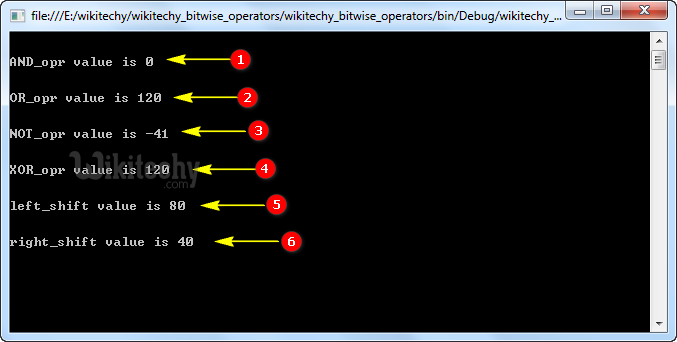1. Here in this output the AND operation variables "a" (value=40) and "b" (value=80) has been shown with its output result as "0".
2. In this output, the OR operation for the variable "a" (value=40) and "b" (value=80) has been shown with its output result as "120".
3. Here in this output the NOT operation for the variable "a" (value=40) and "b" (value=80) has been shown with its output result as "-41".
4. Here in this output the XOR operation for the variable "a" (value=40) and "b" (value=80) has been shown with its output result as "120".
5. Here in this output the Left shift operation for the variable "a" (value=40) has been shown with its output result as "80".
6. Here in this output the Right shift operation for the variable "b" (value=40) has been shown with its output result as "40".